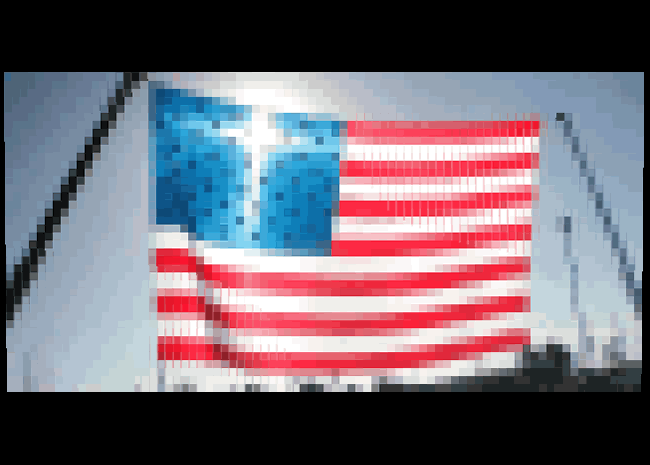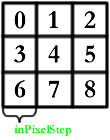Lighthouse3d.comBugsLed Shader Tutorial[Previous] [Next: part II]

# Led Shader Tutorial - Part I

This tutorial was written by Jason Gorski. Do mail him with feedback on your thoughts, comments and suggestions, regarding this great shader effect (jasejc 'at' aol.com).

### Pixelated DisplayThe first thing we must do is transform our texture into one with bigger pixel regions. This is essential for getting that pixelated look of a LED screen. Later we will look at improving this pixelation as well as adding color discretizing effects.

What does this shader require for setup? Not much really. Simply load a texture, set up some uniforms, and send a rectangular quad -- a series of four vertices connected in a rectangular formation -- down the pipeline with some texture coordinates assigned.

The vertex program for this shader is trivial. It simply passes the texture coordinates through to the fragment program and calls a standard ftransform() on the vertex position. It need not be discussed any further. The important stuff all takes place in the fragment program.

Uniforms used in the fragment program:
• int "pixelSize" - size of bigger "pixel regions" in screen pixel units. The lower this is, the closer to the original picture you will get.
• vec2 "textureSize" - two floats for the width and height of our texture. You'll want the width and height to both be evenly divisable by the pixelSize so that you don't have pixel regions cut short by the edge of the texture.
• sampler2D "texture" - a reference to the texture to be rendered.

Take a look at the diagram and description below for a better understanding of these variables and how they work in the program.As denoted by the legend in the lower left corner of the figure, the text in green represents numbers or variables in texture coordinate space and those in blue refer to pixel coordinate space. As you can see, "textureSize" is a vector containing the size of the texture in pixels. "pixelSize" is the size of the bigger pixel regions we will be creating. These bigger pixel regions have width = height in pixel coordinates because they are squares. Therefore, only one value is necessary to be passed to the shader. So these bigger pixel regions are made up of actual pixels right? Yes. So what we are going to do is take a reasonable sample of the pixels contained in our pixel region. I say reasonable because it is a fixed sampling of 9 pixels aranged in a 3x3 square configuration. The advantage to this method of sampling is that the time it takes to render the LED display does not increase the larger you make these pixel regions. This is a nice property to have.

To understand this point further you must think in parallel terms. Each fragment of the LED display is being sent to the pixel shader. So each fragment must determine which pixel region it is in and then sample that region to get its color. We could calculate the average of all the pixels in our pixel region but consider this carefully. Each fragment would have to do these calculations! So the bigger we made our pixel regions the more calculations each fragment would have to do. So instead we take a fixed sampling of our region.

Look at the enlarged pixel region shown in the diagram. This illustrates how we do our sampling. Each dot represents a location in the pixel region from which we will take some color. We simply average all the colors we get in this manner to give us the color for the region.

Lets look at some shader code, starting with our non-uniform variables:

``````
vec2 texCoords[KERNEL_SIZE];
vec4 avgColor;
vec2 texCoordsStep = 1.0/(vec2(float(textureSize.x),float(textureSize.y))/float(pixelSize));
vec2 pixelBin = floor(gl_TexCoord.st/texCoordsStep);
vec2 inPixelStep = texCoordsStep/3.0;
vec2 inPixelHalfStep = inPixelStep/2.0;
```
```

• texCoords - stores 9 offsets from the base case shown in the diagram (the lower left pixel region of our texture).
• avgColor - It will hold our final color which will be an average of our 9 sampled points.
• texCoordsStep - holds the width and height, in texture coordinates, of our pixel regions. Although the pixel regions are square these two values will likely be different due to the fact that we are now talking about texture coordinate space not pixel space. Since the texture coordinates range from 0.0 to 1.0 in both the x and y directions and our texture defined in pixels is likely different in the x and y directions, we get different texCoordsSteps.
• pixelBin - tells us which pixel region we are in relative to the base case. This helps us in computing our offsets from the base case when we do our texture lookups.
• inPixelStep, inPixelHalfStep - as shown in the diagram, these values hold x and y offsets, in texture coordinates, from the lower left corner of our pixel region. We use these to pinpoint the exact location of our texture lookups (the dots in the diagram).

So lets try and understand further how we are getting our texCoords by looking at more code:

``````
float offset = pixelBin * texCoordsStep;
texCoords = vec2(inPixelHalfStep.x, inPixelStep.y*2.0 + inPixelHalfStep.y) + offset;
texCoords = vec2(inPixelStep.x + inPixelHalfStep.x, inPixelStep.y*2.0 + inPixelHalfStep.y) + offset;
texCoords = vec2(inPixelStep.x*2.0 + inPixelHalfStep.x, inPixelStep.y*2.0 + inPixelHalfStep.y) + offset;
texCoords = vec2(inPixelHalfStep.x, inPixelStep.y + inPixelHalfStep.y) + offset;
texCoords = vec2(inPixelStep.x + inPixelHalfStep.x, inPixelStep.y + inPixelHalfStep.y) + offset;
texCoords = vec2(inPixelStep.x*2.0 + inPixelHalfStep.x, inPixelStep.y + inPixelHalfStep.y) + offset;
texCoords = vec2(inPixelHalfStep.x, inPixelHalfStep.y) + offset;
texCoords = vec2(inPixelStep.x + inPixelHalfStep.x, inPixelHalfStep.y) + offset;
texCoords = vec2(inPixelStep.x*2.0 + inPixelHalfStep.x, inPixelHalfStep.y) + offset;
```
```

Each of these offsets coorespond to one of the dots in the pixel region shown earlier. Figure 1 below shows which offset goes to which dot:Figure 1Figure 2 Looking at Figure 1 we can see that to get to the center of box 0 we must step by "inPixelHalfStep.x" in the x direction and "inPixelStep.y*2.0 + inPixelHalfStep.y" in the y direction. To get to the center of box 1 we must step by "inPixelStep.x + inPixelHalfStep.x" in the x direction and "inPixelStep.y*2.0 + inPixelHalfStep.y" in the y direction and so on... These offsets only tell us how to get to our sample points in our base case (the lower left corner of our texture). Next we must apply another offset that will get us to the cooresponding sample spots in the particular pixel region of the current fragment. This is why we add "pixelBin * texCoordsStep" to each offset. It puts us into the correct pixel region bin. Figure 2 helps illustrate this point. See how "pixelBin" helps us step from this base case to a different pixel region?

Conceptual summary of shader steps for each fragment (The current fragment being processed is referred to in the first person):

• compute base case sample locations in texture coordinates
• find out which pixel region I am in and apply that offset to the base case to get my sample locations
• use texture coordinates I have computed to get 9 color values from my pixel region
• I get assign the average of those colors

Go to LED Shader Display Tutorial - Part II for a look at improving this technique!

### Advanced Topic Section This section is not needed to understand the tutorial.

Why work with texture coordinates from the beginning?
You may be wondering why I have chosen to do all these offsets completely in texture coordinates. I mean, conceivably if we did our offsets in pixel coordinates first and then convert to texture coordinates just before doing our lookups we could save computation on the offsets because our width and height would be equal. Admitedly this could be a better option. In fact, when I later considered how I could implement transitions between textures as well as some animations it became apparent that this option might eventually be needed to prevent the pixel regions from moving when animating the texture coordinates from OpenGL. But, in order to get the x and y coordinates of each fragment we would need to add a varying vector to our shader to transfer x and y coordinates from our vertex program to our fragment program since this information is not readily available through GLSL's built in fragment attributes. This would be relatively trivial if we knew our billboard existed on the xy-plane. However, what if it doesn't. What if it has been translated, rotated, and/or scaled. Well now it's not so trivial. The computation to figure out x and y coordinates in a non-standard coordinate plane is not easy. It is probably still a better route since this computation only has to be calculated four times (once for each vertex in the quad). It is a consideration of mine for possible future improvements to this shader.

The code (if you are going to use the code you should refer to the Source and Demo page):

``````

void main (void)
{
gl_TexCoord = gl_MultiTexCoord0;
gl_Position = ftransform();
}

/*************************************************************
*Email: jasejc 'at' aol.com
*CS594 University of Illinios at Chicago
*
*************************************************************/

#define KERNEL_SIZE 9

//size of bigger "pixel regions". These regions are forced to be square
uniform int pixelSize;

//dimensions in pixels of billboardTexture
uniform ivec2 billboardSize;

//texure to be applied to billboard quad
uniform sampler2D billboardTexture;

vec2 texCoords[KERNEL_SIZE]; //stores texture lookup offsets from a base case

void main(void)
{
//will hold our averaged color from our sample points
vec4 avgColor;

//width of "pixel region" in texture coords
vec2 texCoordsStep = 1.0/(vec2(float(billboardSize.x),float(billboardSize.y))/float(pixelSize));

//"pixel region" number counting away from base case
vec2 pixelBin = floor(gl_TexCoord.st/texCoordsStep);

//width of "pixel region" divided by 3 (for KERNEL_SIZE = 9, 3x3 square)
vec2 inPixelStep = texCoordsStep/3.0;
vec2 inPixelHalfStep = inPixelStep/2.0;

//use offset (pixelBin * texCoordsStep) from base case
// (the lower left corner of billboard) to compute texCoords
float offset = pixelBin * texCoordsStep;

texCoords = vec2(inPixelHalfStep.x, inPixelStep.y*2.0 + inPixelHalfStep.y) + offset;
texCoords = vec2(inPixelStep.x + inPixelHalfStep.x, inPixelStep.y*2.0 + inPixelHalfStep.y) + offset;
texCoords = vec2(inPixelStep.x*2.0 + inPixelHalfStep.x, inPixelStep.y*2.0 + inPixelHalfStep.y) + offset;
texCoords = vec2(inPixelHalfStep.x, inPixelStep.y + inPixelHalfStep.y) + offset;
texCoords = vec2(inPixelStep.x + inPixelHalfStep.x, inPixelStep.y + inPixelHalfStep.y) + offset;
texCoords = vec2(inPixelStep.x*2.0 + inPixelHalfStep.x, inPixelStep.y + inPixelHalfStep.y) + offset;
texCoords = vec2(inPixelHalfStep.x, inPixelHalfStep.y) + offset;
texCoords = vec2(inPixelStep.x + inPixelHalfStep.x, inPixelHalfStep.y) + offset;
texCoords = vec2(inPixelStep.x*2.0 + inPixelHalfStep.x, inPixelHalfStep.y) + offset;

//take average of 9 pixel samples
avgColor = texture2D(billboardTexture, texCoords) +
texture2D(billboardTexture, texCoords) +
texture2D(billboardTexture, texCoords) +
texture2D(billboardTexture, texCoords) +
texture2D(billboardTexture, texCoords) +
texture2D(billboardTexture, texCoords) +
texture2D(billboardTexture, texCoords) +
texture2D(billboardTexture, texCoords) +
texture2D(billboardTexture, texCoords);

avgColor /= float(KERNEL_SIZE);